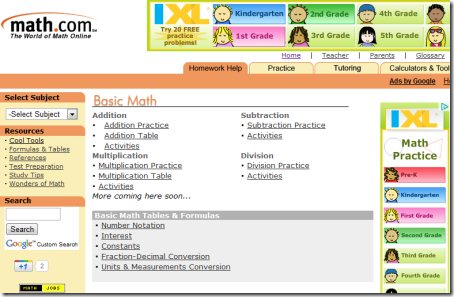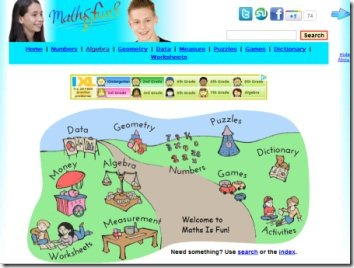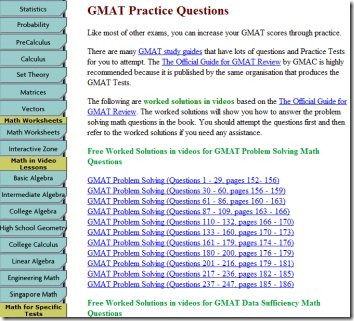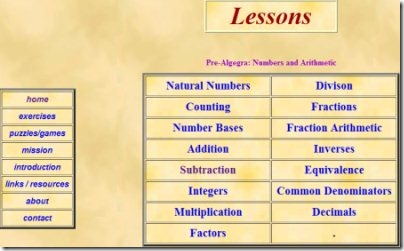Editor Ratings:
User Ratings:
[Total: 0 Average: 0]

Here are 8 free Maths learning websites for those who hate it. Yea..you read it right, these Math websites are indeed for those who hate mathematics and have to spend a couple of hours daily for learning Maths. These websites will help them make Maths fun, basically help them with learning Maths easily, interactive Math videos, Maths quiz, Maths worksheets, Mathematics tricks,  Math Puzzles, cool Math games and more.

We can not deny the fact that mastering Maths facts is extremely important for students as they learn arithmetic, geometry, trigonometry, and other such chapters. Maths, sometimes become very boring for students, at least for my younger mischievous brother. Hence, I made my mind to look for some Math websites that deliver learning Maths with fun including cool Math games, Maths quiz, Math videos, and Maths worksheets.

Once I found some of the free Maths learning websites, I decided to share them with my readers too. Lets get into details of each Math website.

## Math.com

Math.com is one of the most popular websites that helps you to learn Maths online for free. This free Math website comes up with various navigational subjects in Maths such as Basic Math, Algebra, Trigonometry, Statistics, Calculus and a few more. Just select a category you want to learn or practice.In addition, Math.com also provide practice worksheets for each subject, different types of calculators & tools, lets you play cool math games, and more. Learning Maths is so easy now..Go ahead and make Maths fun!

## MathisFun

MathisFun is another popular Math website that provides all important Maths chapters to learn & play around with such as Numbers, Algebra, Geometry, Data, Money, and Measurement. You also play cool Math games, Math puzzles, do Math worksheets, and various other activities.Under each important topic, there are full-fledged chapters that cover a selected topic. For instance: If you click on Algebra, it will take you to a page where you will find all the chapters related to Algebra, right from very basic algebra to advanced algebra. Go ahead and learn Maths with fun..!

Also check out free Maths learning Software reviewed by us.

## Interactive Mathematics

IntMath is yet another excellent Math website that covers almost all Maths chapters to help you learn & understand Maths easily. This one is a full-fledged Math website that has Units such as Algebra, Money, Graphs, Trigonometry, Complex, Probability, Differentiation, Integration, Higher calculus.Under each Unit, given are various chapters. For Example: Algebra includes chapters such as Numbers, Basic Algebra, Quadratic Equations, Matrices & Determinants, Inequalities and more. Similarly, Differentiation is a separate Unit that includes chapters such as Introduction to Calculus, Differentiation, Applications of Differentiation, and the like.

In short, Interactive Mathematics has been developed for all grades students. Go ahead and master all Maths facts..!

## Online Math Learning

OnlineMathLearning is one more Math website that helps you with learning Maths for free. This one is complete Maths tool in the sense that apart from basic Mathematics, this free Math website provides Maths up to CAT, GMAT level. You can prepare for all competitive Maths exams such as SAT Math, ACT Math, GMAT Math, Engineering Math and more.In addition, OnlineMathLearning also provides fun while learning Maths. You can play cool Math games, Math Trivia, Fun Games and much more. Go ahead and prepare for all competitive exams while having fun..!

## Math-Videos-Online

Math-Videos-Online is an excellent online Maths learning service that brings you Math Videos for all the Maths Units. There are various Math Videos such as Algebra videos, Geometry videos, Probability Videos, and much more. Under each Unit, given are Videos for each & every chapter/topic.

Math Videos Online is a must for each & every Maths learner. The Maths Video tutorials are absolutely amazing that helps you to learn Maths easily. It explains each step of solving a Maths equation. Go ahead and understand Maths with these Math Videos. Go ahead and watch these videos for understanding Maths in a better way..!

## Visual Math Learning

Visual Math Learning is another Math website that helps you in learning Maths free. The navigation tab consists of Math Lessons, Math Exercises, Maths Puzzles/ Math Games, Introduction and a few more categories that will make you understand each & every Math Unit.This free Website for learning Maths is extremely beneficial if you practice regularly. Go ahead and logon to Visual Math Learning free..!

While learning Maths, you might also like to test your IQ level. Do check out Online IQ Test Websites reviewed by us.

## Math Games

Math Games is a complete fun Maths website that provides learning Maths in a rather entertaining way. This Math website has all cool Math Games such as Arithmetic challenge, Math Test, Monkey Math Balance, Math Mountain, The Math Quiz, Color21 and many more.This Math website has been developed especially for kids who hate solving Maths problem. This website will help them to learn Maths and solve Math problems while playing game. The website will stimulate kids to solve Math Puzzle, resulting into master Maths facts. Go ahead and play all cool Math games free..!

## Easy Calculation

Ending my short post with this last but of course not the least Math website, Easy Calculation. This free Math website is extremely beneficial learning Maths right from the very beginning. Apart from providing the regular Math Lessons, Easy Calculation also serves you with Interesting Math facts, Maths tricks, Ancient Numerals, Unit conversion, Math dictionary and much more.The website has been developed especially for higher level students who are preparing for competitive Exams. Go ahead and learn easy calculation quickly..!! Students who are preparing for GRE can check out some Websites for GRE preparation reviewed by us.

 Editor Ratings: User Ratings:[Total: 0 Average: 0]